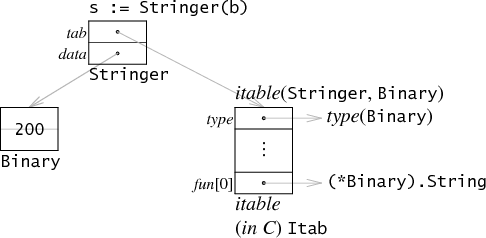# Golang 接口与反射知识要点

## 1. 接口类型变量type Stringer interface {
String() string
}

type Binary uint64

func (i Binary) String() string {
return strconv.Uitob64(i.Get(), 2)
}

func (i Binary) Get() uint64 {
return uint64(i)
}

b := Binary(200)
var s Stringer = b

Go 是静态类型语言（statically typed）。一个接口类型的不同变量总是有同样静态类型，尽管在运行时，接口变量的保存的实际值会改变。下面例子中，无论 r 被赋予的什么实际值，r 的类型总是 io.Reader。

var r io.Reader
r = os.Stdin
r = new(bytes.Buffer)
// and so on

## 2. 类型断言

var r io.Reader
tty, err := os.OpenFile("/dev/tty", os.O_RDWR, 0)
if err != nil {
return nil, err
}
r = tty

var w io.Writer
w = r.(io.Writer)

## 3. 鸭子类型

Go 语言虽然是静态语言，但在接口类型中使用了鸭子类型。不同于其他鸭子类型语言的是，它实现了在编译时进行静态检查，比如变量是否实现接口方法、调用接口方法时参数个数是否相符，同时也不失鸭子类型带来的灵活和自由。

## 4. 反射机制

• 什么是反射机制？

• 使用反射的场景？

Go 语言中使用反射的场景：有时候需要根据某些条件决定调用哪个函数，比如根据用户的输入来决定，但事先无法知道接受到的参数是什么类型，全部以 interface{} 类型接受。这时就需要对函数的参数进行反射，在运行期间动态地执行函数。感兴趣的读者可以参考 fmt.Sprint(a ...interface{}) 方法的源码。

## 5. reflect 包

### TypeOf()、ValueOf()

reflect 包封装了很多简单的方法（reflect.TypeOf 和 reflect.ValueOf）来动态获得类型信息和实际值（reflect.Type，reflect.Value）。

var x float64 = 3.4
fmt.Println("type:", reflect.TypeOf(x))  // 打印 type: float64

fmt.Println("type:", reflect.TypeOf(r))  // 打印 type: *strings.Reader

reflect.TypeOf 方法的函数签名是 func TypeOf(i interface{}) Type 。它接受任意类型的变量。当我们调用 reflect.TypeOf(x) 时，x 首先存储在一个空接口类型中，作为传参。reflect.TypeOf 解析空接口，恢复 x 的类型信息。而调用 reflect.ValueOf 则可以恢复 x 实际值。

var x float64 = 3.4
fmt.Println("value:", reflect.ValueOf(x).String()) // 打印 value: <float64 Value>

### Type()、Kind()

reflect.Type 和 reflect.Value 都提供了很多方法支持来操作他们。1. reflect.Value 的 Type() 方法返回实际类型信息；2. reflect.Type 和 reflect.Value 都有 Kind() 方法，来获得实际值的底层类型，结果对应的是 reflect 包中定义的常量；3. reflect.Value 的那些以类型名为方法名的方法，比如 Int()、Float()，能获得实际值。

var x float64 = 3.4
v := reflect.ValueOf(x)
fmt.Println("type:", v.Type())
fmt.Println("kind is float64:", v.Kind() == reflect.Float64)
fmt.Println("value:", v.Float())

shell script type: float64 kind is float64: true value: 3.4

func main() {
type MyInt int
var x MyInt = 7
v := reflect.ValueOf(x)
fmt.Println("type:", v.Type())
fmt.Println("kind is int:", v.Kind() == reflect.Int)
fmt.Println("value:", v.Int())
}

shell script type: main.MyInt kind is int: true value: 7

v 调用 Kind() 仍是 reflect.Int，即使 x 的静态类型是 MyInt 而不是 int。总而言之，Kind() 方法无法区分来自 MyInt 的整数型和 int 型，但 Type() 方法可以

### Interface()

Interface() 方法能从 reflect.Value 变量中恢复接口值，是 ValueOf() 的逆向。注意的是，Interface() 方法返回总是静态类型 interface{}。

### DeepEqual()

DeepEqual 用于递归地检查元素是否全等

## 6. 反射对象的可设置性

### SetXXX(), CanSet()

var x float64 = 3.4
v := reflect.ValueOf(x)
v.SetFloat(7.1)  // will panic: reflect.Value.SetFloat using unaddressable value

var x float64 = 3.4
v := reflect.ValueOf(x)
fmt.Println("settability of v:", v.CanSet())  // settability of v: false

### Elem()

var x float64 = 3.4
p := reflect.ValueOf(&x) // Note: take the address of x.
fmt.Println("type of p:", p.Type())  // type of p: *float64
fmt.Println("settability of p:", p.CanSet())  // settability of p: false

// Elem returns the value that the interface v contains
// or that the pointer v points to.
// It panics if v's Kind is not Interface or Ptr.
// It returns the zero Value if v is nil.
func (v Value) Elem() Value
v := p.Elem()
fmt.Println("settability of v:", v.CanSet())  // settability of v: true

v.SetFloat(7.1)
fmt.Println(v.Interface())  // 7.1
fmt.Println(x)  // 7.1

## 7. Struct 的反射

### NumField(), Type.Field(i int)

type T struct {
A int
B string
}

t := T{23, "skidoo"}
s := reflect.ValueOf(&t).Elem()
typeOfT := s.Type()

for i := 0; i < s.NumField(); i++ {
f := s.Field(i)
fmt.Printf("%d: %s %s = %v\n", i,
typeOfT.Field(i).Name, f.Type(), f.Interface())
}

Type.Field(i int) 方法返回字段信息，一个 StructField 类型的对象，包含字段名等。

0: A int = 23
1: B string = skidoo

### Value.Field(i int)

T 的字段必须是首字母大写的才可以设置，因为只有暴露的 struct 字段，才具有可设置性

s.Field(0).SetInt(77)
s.Field(1).SetString("Sunset Strip")
fmt.Println("t is now", t) // t is now {77 Sunset Strip}

Value.Field(i int) 返回 struct s 的字段实际值，所以可以用来设置操作。注意 Type.Field(i int) 和 Value.Field(i int) 的用途区别：前者总是负责和实际类型信息获取相关的操作，后者是与实际值相关的操作

## 参考文档

The Laws of Reflection

Go Data Structures: Interfaces

Go 语言的数据结构：Interfaces

posted @ 2019-10-04 03:48  黄挤挤  阅读(...)  评论(...编辑  收藏